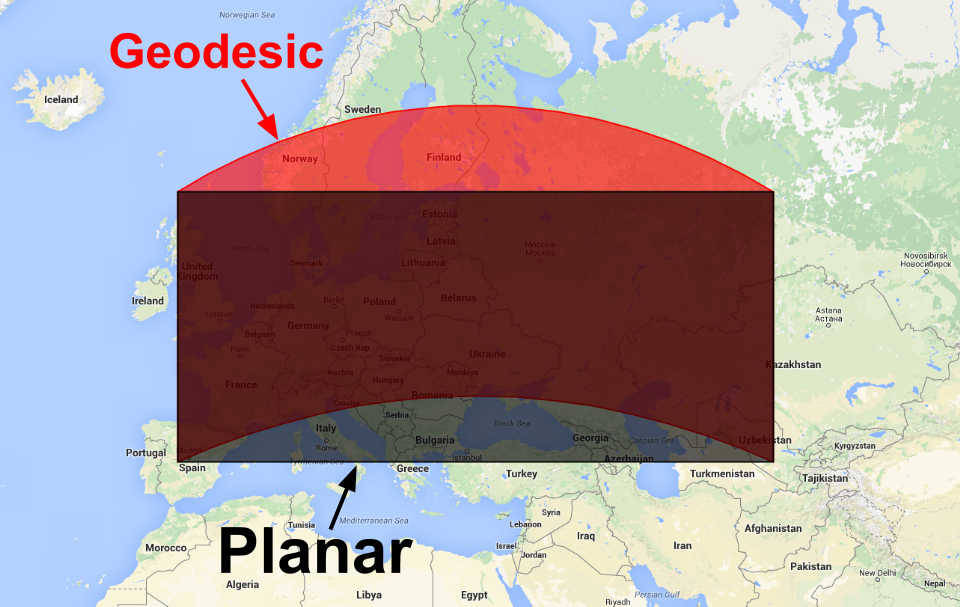Geodesic vs. Planar Geometries

A geometry created in Earth Engine is either geodesic (i.e. edges are the shortest path on the surface of a sphere) or planar (i.e. edges are the shortest path in a 2-D Cartesian plane). No one planar coordinate system is suitable for global collections of features, so Earth Engine's geometry constructors build geodesic geometries by default. To make a planar geometry, constructors have a geodesic parameter that can be set to false:

var planarPolygon = ee.Geometry(polygon, null, false);

Figure 1 shows the difference between the default geodesic polygon and the result of converting the polygon to a planar representation.Figure 1. A geodesic polygon (red) and a planar polygon (black).

You can convert between geodesic and planar geometries using the ee.Geometry constructor.